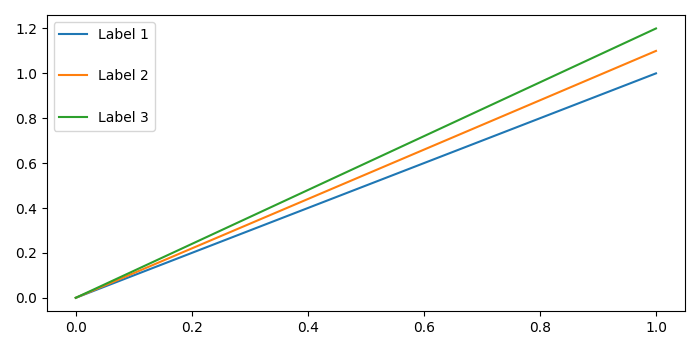# How to adjust the space between legend markers and labels in Matplotlib?

MatplotlibPythonData Visualization

To adjust the space between legend markers and labels, we can use labelspacing in legend method.

## Steps

• Plot lines with label1, label2 and label3.

• Initialize a space variable to increase or decrease the space between legend markers and label.

• Use legend method with labelspacing in the arguments.

• To display the figure, use show() method.

## Example

from matplotlib import pyplot as plt
plt.rcParams["figure.figsize"] = [7.00, 3.50]
plt.rcParams["figure.autolayout"] = True
plt.plot([0, 1], [0, 1.0], label='Label 1')
plt.plot([0, 1], [0, 1.1], label='Label 2')
plt.plot([0, 1], [0, 1.2], label='Label 3')
space = 2
plt.legend(labelspacing=space)
plt.show()

## Output